Get instant live expert help with Excel or Google Sheets“My Excelchat expert helped me in less than 20 minutes, saving me what would have been 5 hours of work!”

#### Post your problem and you’ll get expert help in seconds.

Your message must be at least 40 characters
Our professional experts are available now. Your privacy is guaranteed.

# How to Use the Excel DATE Function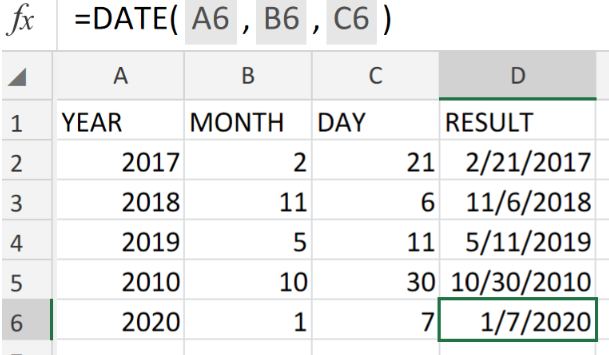Figure 1. Excel Date Function.

If we have to assemble dates that require dynamic changes based on other values in our worksheet, we are going utilize the Excel DATE Function.

## Formula Syntax

`=DATE (year, month, day)`

• day = Number value of the day
• month = Number value of the month
• year = Number value of the year

The DATE Function is the most essential function to understand when it comes to working with dates in Excel.

## How to use the Excel DATE Function.

We are now going to use individual year, month, and day components to create a valid Excel date.

1. List the dates available for conversion in a column of our worksheet.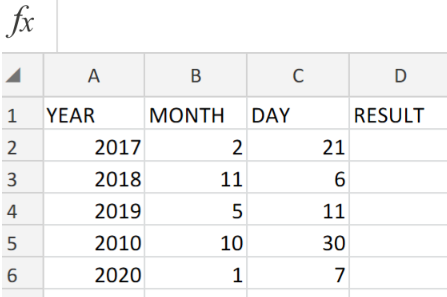Figure 2. Excel Date Function.

Be sure to provide an empty column for Excel to return the dates.

1. In our worksheet example above, the DATE formula we will enter into cell D2 is as follows;

`=DATE(A2,B2,C2)`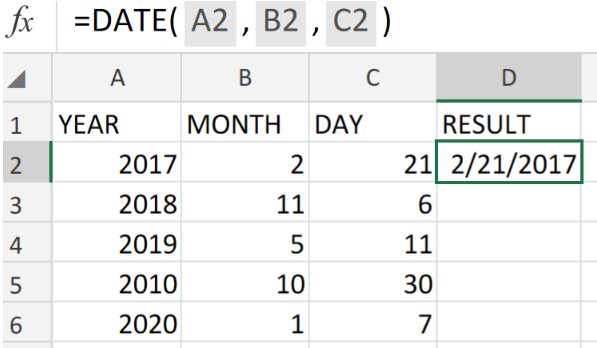Figure 3. Excel Date Function.

Excel returned 2/21/2017 as our required date format.

1. Modify the DATE formula in cell D2 and copy it into the other cells in the RESULT column to achieve the required date format.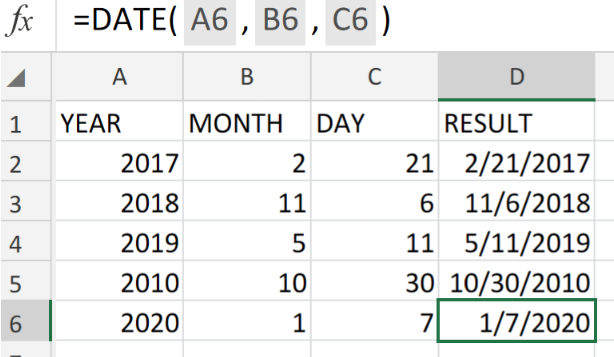Figure 4. Excel Date Function.

other Excel functions such as, the COUNTIFS and SUMIFS Functions cannot always recognize dates entered in the text format.

The DATE Function makes it easier to generate a date using year, month, and day values obtained from a formula result or cell reference.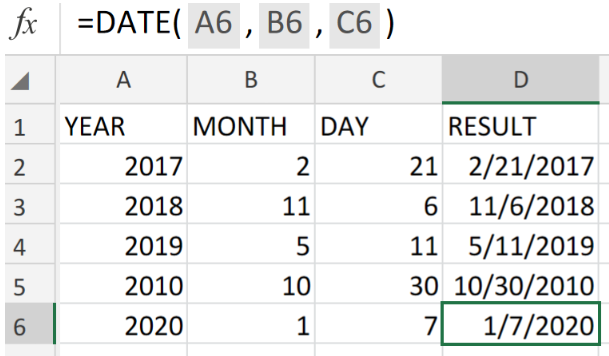Figure 5. Final Result.

## Instant Connection to an Expert through our Excelchat Service:

Our live Excelchat Service is here for you. We have Excel Experts available 24/7 to answer any Excel questions you may have. Guaranteed connection within 30 seconds and a customized solution for you within 20 minutes.

### Did this post not answer your question? Get a solution from connecting with the expert.Another blog reader asked this question today on Excelchat:
Related blogs
Solution examplesI have multiple columns on my spread sheet and want to switch from A-Z to Z-A based on values in column A
Solved by V. H. in 38 minsConcat formula is incorrectly formatting the date in column C. Currently column B has dd/mm/yyyy and I require mm/dd/yyyy
Solved by Z. Y. in 31 minsI am trying to utilize a complicated sumif or sumifs formula that can pull a total amount from a date and a drop down box from another tab.
Solved by E. B. in 15 minsI have a spreadsheet that totals invoices. A bunch of cells at the bottom of the spreadsheet calculates totals based on which department the invoice is charged to. I'd like to add a function where it also considers the date (so if it's an invoice in January, it goes to a January total, February, etc.). But I can't figure out how to write it properly...
Solved by K. J. in 27 minsIf I want to write a formula that states" "If this name shows up K24, and this word shows up in J24 pull this number from M24" I can creat that formula - however I need to drag this down so it pulls from row 24 - 60 - is there way to do this?
Solved by F. D. in 40 mins## Subscribe to Excelchat.coAnother blog reader asked this question today on Excelchat: